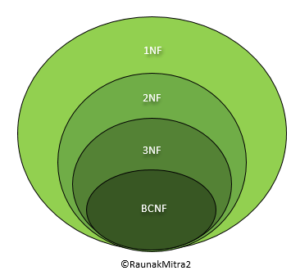Related Articles

# Boyce-Codd Normal Form (BCNF)

• Difficulty Level : Medium
• Last Updated : 27 Jan, 2020

Application of the general definitions of 2NF and 3NF may identify additional redundancy caused by dependencies that violate one or more candidate keys. However, despite these additional constraints, dependencies can still exist that will cause redundancy to be present in 3NF relations. This weakness in 3NF, resulted in the presentation of a stronger normal form called Boyce–Codd Normal Form (Codd, 1974).

Although, 3NF is adequate normal form for relational database, still, this (3NF) normal form may not remove 100% redundancy because of X?Y functional dependency, if X is not a candidate key of given relation. This can be solve by Boyce-Codd Normal Form (BCNF).

Boyce-Codd Normal Form (BCNF):
Boyce–Codd Normal Form (BCNF) is based on functional dependencies that take into account all candidate keys in a relation; however, BCNF also has additional constraints compared with the general definition of 3NF.

A relation is in BCNF iff, X is superkey for every functional dependency (FD) X?Y in given relation.

In other words,

A relation is in BCNF, if and only if, every determinant is a Form (BCNF) candidate key.

Note – To test whether a relation is in BCNF, we identify all the determinants and make sure that they are candidate keys.The Normal Form Hierarchy

You came across a similar hierarchy known as Chomsky Normal Form in Theory of Computation. Now, carefully study the hierarchy above. It can be inferred that every relation in BCNF is also in 3NF. To put it another way, a relation in 3NF need not to be in BCNF. Ponder over this statement for a while.
To determine the highest normal form of a given relation R with functional dependencies, the first step is to check whether the BCNF condition holds. If R is found to be in BCNF, it can be safely deduced that the relation is also in 3NF, 2NF and 1NF as the hierarchy shows. The 1NF has the least restrictive constraint – it only requires a relation R to have atomic values in each tuple. The 2NF has a slightly more restrictive constraint.
Read this for a clear understanding of 2NF
The 3NF has more restrictive constraint than the first two normal forms but is less restrictive than the BCNF. In this manner, the restriction increases as we traverse down the hierarchy.

Example-1:
Find the highest normal form of a relation R(A, B, C, D, E) with FD set as:

`{ BC->D, AC->BE, B->E } `

Explanation:

• Step-1: As we can see, (AC)+ ={A, C, B, E, D} but none of its subset can determine all attribute of relation, So AC will be candidate key. A or C can’t be derived from any other attribute of the relation, so there will be only 1 candidate key {AC}.
• Step-2: Prime attributes are those attribute which are part of candidate key {A, C} in this example and others will be non-prime {B, D, E} in this example.
• Step-3: The relation R is in 1st normal form as a relational DBMS does not allow multi-valued or composite attribute.

The relation is in 2nd normal form because BC->D is in 2nd normal form (BC is not a proper subset of candidate key AC) and AC->BE is in 2nd normal form (AC is candidate key) and B->E is in 2nd normal form (B is not a proper subset of candidate key AC).

The relation is not in 3rd normal form because in BC->D (neither BC is a super key nor D is a prime attribute) and in B->E (neither B is a super key nor E is a prime attribute) but to satisfy 3rd normal for, either LHS of an FD should be super key or RHS should be prime attribute. So the highest normal form of relation will be 2nd Normal form.

Note –A prime attribute cannot be transitively dependent on a key in BCNF relation.

Consider these functional dependencies of some relation R,

AB ->C
C ->B
AB ->B

Suppose, it is known that the only candidate key of R is AB. A careful observation is required to conclude that the above dependency is a Transitive Dependency as the prime attribute B transitively depends on the key AB through C. Now, the first and the third FD are in BCNF as they both contain the candidate key (or simply KEY) on their left sides. The second dependency, however, is not in BCNF but is definitely in 3NF due to the presence of the prime attribute on the right side. So, the highest normal form of R is 3NF as all three FD’s satisfy the necessary conditions to be in 3NF.

Example-2:
For example consider relation R(A, B, C)

```A -> BC,
B -> A```

A and B both are super keys so above relation is in BCNF.

Note –
BCNF decomposition may always not possible with dependency preserving, however, it always satisfies lossless join condition. For example, relation R (V, W, X, Y, Z), with functional dependencies:

```V, W -> X
Y, Z -> X
W -> Y ```

It would not satisfy dependency preserving BCNF decomposition.
Note -:Redundancies are sometimes still present in a BCNF relation as it is not always possible to eliminate them completely.

Attention reader! Don’t stop learning now.  Practice GATE exam well before the actual exam with the subject-wise and overall quizzes available in GATE Test Series Course.

Learn all GATE CS concepts with Free Live Classes on our youtube channel.

My Personal Notes arrow_drop_up